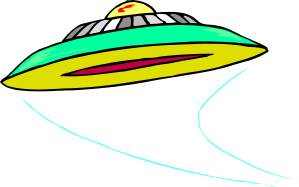# Exam-Style Questions on Logarithms

## Problems on Logarithms adapted from questions set in previous Mathematics exams.

### 1.

IB Standard

Find the value of the following:

(a) $$log_464$$;

(b) $$log_7\frac17$$;

(c) $$log_{25}5$$;

(d) Use the solutions to the previous parts of this question to help solve:

$$log_464+log_7\frac17-log_{25}5=log_4x$$

### 2.

IB Standard

(a) $$\log _5 25$$

(b) $$\log _6 3 + \log _6 12$$

(c) $$\log _2 12 - \log _2 6$$

### 3.

IB Standard

Find the value of

(a) $$\log _4 2 + \log _4 8$$

(b) $$\log_2 60-\log_2 15$$

(c) $$27^{\log_3 4}$$

### 4.

IB Applications and Interpretation

In an old science fiction book the author described the intensity of reverse polarity, $$P$$ measured in treckons, is a function of the nebula thrust, $$N$$ measures in whovians. The intensity level is given by the following formula.

$$P = 7 \log_{10}(N \times 10^{8}), N \ge 0$$

(a) An space shuttle has a nebula thrust of $$9.1 × 10^{-3}$$ whovians. Calculate the intensity level, $$P$$ of the shuttle.

(b) A different space shuttle has an intensity level of 112 trekons. Find its nebula thrust, $$N$$.### 5.

IB Standard

(a) Solve $$4x^2 - 8x - 5 = 0$$

(b) Hence solve $$4 \times 25^x - 8 \times 5^x = 5$$

### 6.

IB Analysis and Approaches

(a) Show that $$\log_4 (\sin 2x +2) = \log_2 \sqrt{\sin 2x + 2 }$$

(b) Hence or otherwise solve $$\log_2 (2 \cos x) = \log_4 (\sin 2x + 2)$$ to show that $$x = \frac12 \tan^{-1} 2$$.

### 7.

IB Standard

An arithmetic sequence has $$u_1 = \log_h(j)$$ and $$u_2 = \log_h(jk)$$, where $$h > 1$$ and $$j, k \gt 0$$.

(a) Show that the common difference, $$d = \log_h(k)$$.

(b) Let $$j = h^5$$ and $$k = h^7$$. Find the value of $$\sum_{n=1}^{16} u_n$$.

### 8.

IB Standard

Consider the function $$f (x) = \log_p(24x - 18x^2)$$ , for $$0 \lt x \lt 1$$, where $$p \gt 0$$.

The equation $$f (x) = 3$$ has exactly one solution. Find the value of $$p$$.

If you would like space on the right of the question to write out the solution try this Thinning Feature. It will collapse the text into the left half of your screen but large diagrams will remain unchanged.

The exam-style questions appearing on this site are based on those set in previous examinations (or sample assessment papers for future examinations) by the major examination boards. The wording, diagrams and figures used in these questions have been changed from the originals so that students can have fresh, relevant problem solving practice even if they have previously worked through the related exam paper.

The solutions to the questions on this website are only available to those who have a Transum Subscription.

Exam-Style Questions Main Page

Search for exam-style questions containing a particular word or phrase:

To search the entire Transum website use the search box in the grey area below.